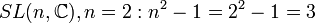# Special linear group:SL(2,C)

(Redirected from SL(2,C))
View a complete list of particular groups (this is a very huge list!)[SHOW MORE]

## Definition

The group$SL(2,\mathbb{C})$ is defined as the group of$2 \times 2$ matrices with entries from the field of complex numbers and determinant$1$, under matrix multiplication.$SL(2,\mathbb{C}) := \left \{ \begin{pmatrix} a & b \\ c & d \\\end{pmatrix} \mid a,b,c,d \in \mathbb{C}, ad - bc = 1 \right \}$.

It is a particular case of a special linear group over complex numbers, special linear group of degree two, and hence of a special linear group.

## Arithmetic functions

Function Value Similar groups Explanation
order of a group cardinality of the continuum The cardinality is at least that of the continuum, because we can inject$\mathbb{C}$ into this group by$x \mapsto \begin{pmatrix} 1 & x \\ 0 & 1 \\\end{pmatrix}$. On the other hand, it is a subset of$\mathbb{C}^4$, so the cardinality is not more than that of the continuum.
exponent of a group infinite there exist elements, such as$\begin{pmatrix} 1 & 1 \\ 0 & 1 \\\end{pmatrix}$, of infinite order.
composition length 2 groups with same composition length Center is simple (isomorphic to cyclic group:Z2) and the quotient group PSL(2,C) is also simple.
chief length 2 groups with same chief length Similar reason to composition length.
dimension of an algebraic group 3 groups with same dimension of an algebraic group As$SL(n,\_), n = 2: n^2 - 1 = 2^2 - 1 = 3$
dimension of a complex Lie group 3 groups with same dimension of a complex Lie group As$SL(n,\mathbb{C}), n = 2: n^2 - 1 = 2^2 - 1 = 3$
dimension of a real Lie group 6 groups with same dimension of a real Lie group Twice its dimension as a complex Lie group.

## Group properties

### Abstract group properties

Property Satisfied? Explanation
abelian group No
nilpotent group No
solvable group No
quasisimple group Yes special linear group is quasisimple (with a couple of finite exceptions). Its inner automorphism group, which is projective special linear group:PSL(2,C), is simple.
simple non-abelian group No The center is$\pm I$, so is proper and nontrivial.

### Topological/Lie group properties

Property Satisfied? Explanation
connected topological group Yes It is generated by matrices of the form$\begin{pmatrix} 1 & x \\ 0 & 1 \\\end{pmatrix}, x \in \R$ and$\begin{pmatrix} 1 & 0 \\ x & 1 \\\end{pmatrix}, x \in \mathbb{C}$. Both sets are connected sets are matrices containing the identity, so the group is connected.
compact group No It contains matrices of the form$\begin{pmatrix} 1 & x \\ 0 & 1 \\\end{pmatrix}, x \in \mathbb{C}$ where the$x$ can be arbitrarily large, so is not compact as a subset of$\mathbb{C}^4$.

## Elements

Further information: element structure of special linear group:SL(2,C)

## Linear representation theory

Further information: linear representation theory of special linear group:SL(2,C)